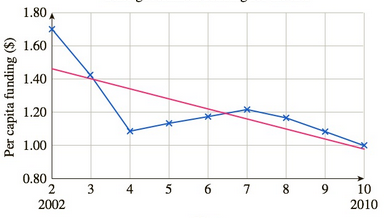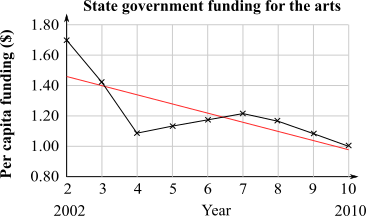Chapter 10.4, Problem 37EFinite Mathematics and Applied Cal...

7th Edition
Stefan Waner + 1 other
ISBN: 9781337274203

Solutions

Chapter
SectionFinite Mathematics and Applied Cal...

7th Edition
Stefan Waner + 1 other
ISBN: 9781337274203
Textbook Problem

Funding for the Arts State governments in the United States spend between $1 and$2 per person on the arts and culture each year. The following chart shows the data for 2002–2010, together with the regression line:44State government funding for the artsYeara. Over the period [ 2 , 6 ] the average rate of change of state government funding for the arts was(A) less than(B) greater than(C) approximately equal to the rate predicted by the regression line.b. Over the period [ 3 , 10 ] the average rate of change of state government funding for the arts was(A) less than(B) greater than(C) approximately equal to the rate predicted by the regression line.c. Over the period [ 4 , 8 ] the average rate of change of state government funding for the arts was(A) less than(B) greater than(C) approximately equal to the rate predicated by the regression line.b. Estimate, to two significant digits, the average rate of change of per capita state government funding for the arts over the period [ 2 , 10 ] . (Be care full to state the unit of measurement.) How does it compare to the slope of the regression line?

(a)

To determine

The average rate of change of the state government funding for the arts during the period [2,6] if the state government spends between $1 and$2 per person on the arts and culture each year from the following options:

A) less than

B) greater than

C) approximately equal to the rate predicted by the regression line.

The following graph shows the data for 20022010, along-with the regression line.Explanation

Given Information:

Consider that the state government spends between $1 and$2 per person on the arts and culture each year.

The following graph shows the data for 20022010, along-with the regression line.

Consider that the state government spends between $1 and$2 per person on the arts and culture each year.

Let the average rate of change of state government funding over the interval [2,6] be the slope of the line in the graph joining the corresponding points on the graph and let it be represented by g.

Let the regression line given in the graph be represented by the function f.

The average rate of change for the regression line over the interval [2,6] is:

Average rate of change=f(6)f(2)62

From the graph, f(6)=1.21,f(2)=1.45

Substitute the value of f(6)=1.21,f(2)=1.45:

f(6)f(2)62=1.211

(b)

To determine

The average rate of change of the state government funding for the arts during the period [3,10] if the state government spends between $1 and$2 per person on the arts and culture each year from the following options:

A) less than

B) greater than

C) approximately equal to the rate predicted by the regression line.

The following graph shows the data for 20022010, along-with the regression line.(c)

To determine

The average rate of change of the state government funding for the arts during the period [4,8] if the state government spends between $1 and$2 per person on the arts and culture each year from the following options:

A) less than

B) greater than

C) approximately equal to the rate predicted by the regression line.

The following graph shows the data for 20022010, along-with the regression line.(d)

To determine

The average rate of change of the change per capita state government funding for the arts over the period [2,10] and then compare it to the slope of the regression line if the state government spends between $1 and$2 per person on the arts and culture each year. The following graph shows the data for 20022010, along-with the regression line.Still sussing out bartleby?

Check out a sample textbook solution.

See a sample solution

The Solution to Your Study Problems

Bartleby provides explanations to thousands of textbook problems written by our experts, many with advanced degrees!

Get Started

Find more solutions based on key concepts